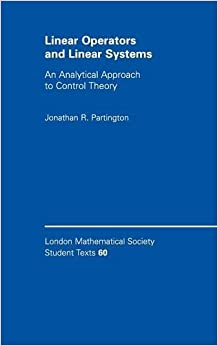# Linear Operators and Linear Systems: An Analytical Approach to Control Theory (London Mathematical Society Student Texts, Series Number 60)

#### Tag: Embedded Systems

Posted on 2021-05-16, by phaelx.

DescriptionDate: 2004
Pages: 178
ISBN: 0521837340
Author: Jonathan R. Partington

Size: 1.2 MB
Format: pdf
This volume presents an introduction to the common ground between operator theory and linear systems theory. Pure mathematical topics are included such as Hardy spaces, closed operators, the gap metric, semigroups, shift-invariant subspaces, the commutant lifting theorem and almost-periodic functions, which would be suitable for a course in functional analysis. The book also includes applications to partial differential equations, the stability and stabilization of linear systems, power signal spaces, and delay systems, treated from an input/output point of view.
Abstract Algebra (Books),Differential Equations (Books),Algebra & Trigonometry

6931 dl's @ 2132 KB/s
9804 dl's @ 2777 KB/s
6609 dl's @ 2143 KB/s

Search More...
Linear Operators and Linear Systems: An Analytical Approach to Control Theory (London Mathematical Society Student Texts, Series Number 60)Related Books